# Understanding a few parts of an array-entered formula in Excelon
Hello,

Can someone provide an explanation for a couple of parts of the array-entered Excel formula described below?

I've been tinkering with an array formula for several days, trying to understand how it does what it does as the solution to the thread located here:
Extract certain items from one list to create another list in Excel

A shortened version (10 rows instead of 20 rows) of the formula is:
``````{=IFERROR(INDEX(B\$1:B\$13,SMALL(IF((C\$4:C\$13=F\$2)*ROW(C\$4:C\$13)=0,1E+38,(C\$4:C\$13=F\$2)*ROW(C\$4:C\$13)),ROWS(\$4:4))),"")}
``````
The purpose of the formula is depicted in the following screenshot where its function is to create an ordered list (in column F) of the names in column B which have an adjacent letter in column C that matches a manually-entered letter in the box at cell F2. (By the way, column E contains a formula which displays numbering only if the adjacent column-F cell is not blank — see footnote *a).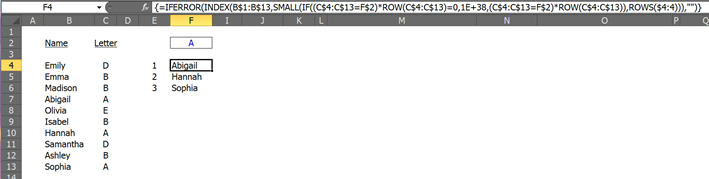(Note: all screenshots in this post are taken from the attached Excel file.)

The formula works great but I really want to understand each part of what it's doing because I have several other places where it would be useful. So working from the inside out, I looked at the cell-display results of various segments when they are entered 1) as standard formulas and 2) when they are entered as array formulas (Ctrl+Shift+Enter).

I also used a trick I learned a couple of years ago which enables you to 3) see the complete results of a formula by selecting the formula in the Formula Bar then pressing F9 (see footnote *b).

The following screenshot shows these three results for several segments of the formula:

• #1 & #2 are shown in column F and
• #3 (the F9 result), is pasted with a black font in column H: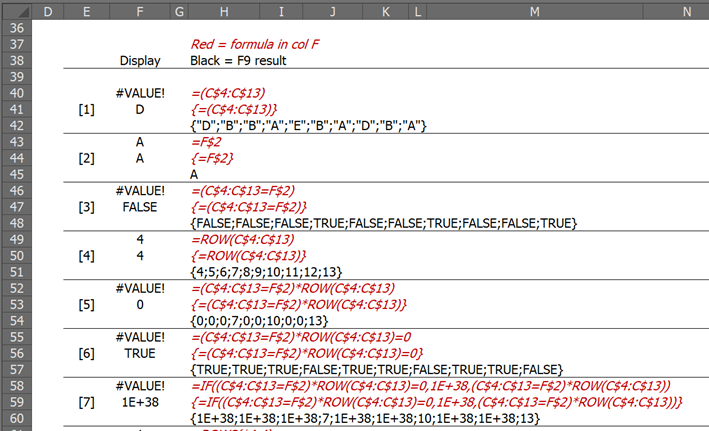By the way, cells with red italics use the Excel4 function =GET.CELL(6, [ref]) to display the formula residing two cells to the left (eg H40 shows the formula in F40) (see footnote *c).

The next thing I did was to transpose the F9 results into a table with heading numbers corresponding to the evaluated segments and wrote down definitions/explanations (blue font) of what I think is happening. These steps are shown in the next two screenshots: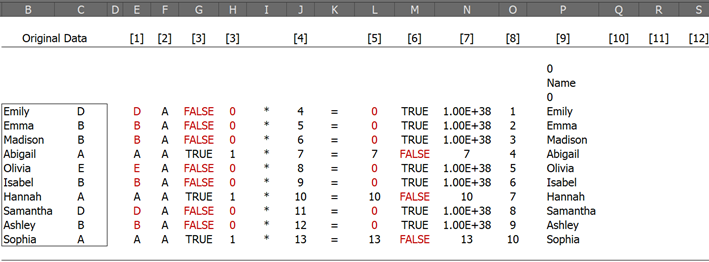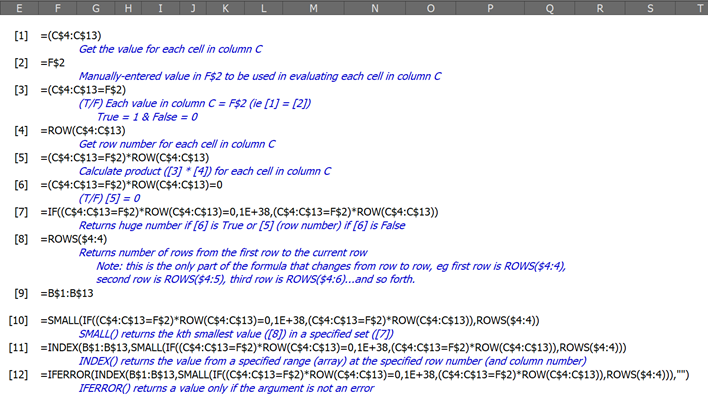I think I understand most of the process up through item #7 and I'm fairly familiar with the INDEX() & IFERROR() functions (in #11 & #12). But I have not previously used the ROWS() or SMALL() functions — although I've read about them and gone through some examples and they seem to be quite straightforward.

I suspect the biggest hurdle is understanding how to get from essentially evaluating each original-data row one-at-a-time to get an array of 10 results at each step (if that's the correct terminology) to having the three names (Abigail, Hannah, & Sophia) which are "still standing" after all the steps (ie do not get assigned the huge number in #7), compiled into the final list of three names.

Maybe if someone could respond with how you would edit/fill-in the blue-font descriptions for #8, #9, & #10, that would do the trick. Other similar examples are always helpful as well.

Thanks, Steve
__________________________________________________

Footnotes:

*a — The formula in column E is:

=IFERROR(IF(OFFSET(E4,0,1)<>"",OFFSET(E4,-1,0)+1,""),"")

pasted down to row 13.

*b — I got the F9 tip from Rory Archibald about two years ago and here is the Microsoft doc on the same topic.

Also, Ctrl+z undoes the function (if you remember to do it before leaving the Formula Bar).

*c — Here is a link I found with some information about Excel4 functions. I'm interested in finding other/better references on this topic in case anyone knows of one.

By the way, the:

=GET.CELL(6, [ref])

function does not, for some reason, display curly brackets when an array formula is present, so I manipulated it a bit to make it work in those cases:

="{"&GET.CELL(6,AutoFilter!H16)&"}"

This is shown in the Name Manager where I named the standard function "Formula" and the manipulated form "ArrayFormula" as shown in the next two screenshots: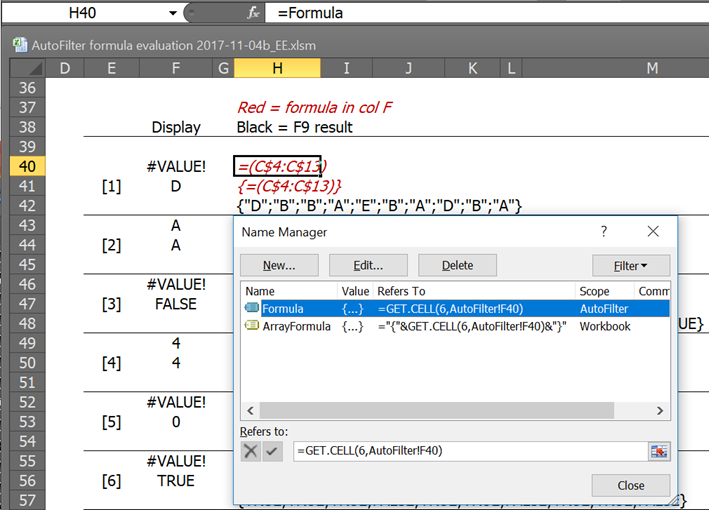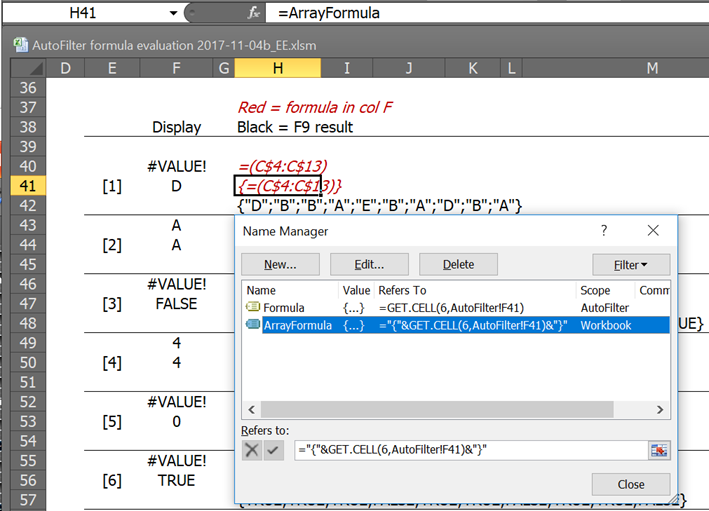File: AutoFilter-array-formula-evaluation.xlsm
Comment
Watch Question

Do more withEXPERT OFFICE® is a registered trademark of EXPERTS EXCHANGE®
Mechanical Engineer
Most Valuable Expert 2013
Top Expert 2013
Commented:
Now that most people use Excel 2010 or later, I really like using the AGGREGATE function instead of SMALL or LARGE for these kind of problems. The advantage is that AGGREGATE doesn't need to be array-entered and can ignore error values. As a result, AGGREGATE doesn't need the IF function to build an array of row numbers that satisfy the criterion of column C equaling the value in F2.

The shortened formula using AGGREGATE does not need to be array-entered.
``````=IFERROR(INDEX(B\$1:B\$13,AGGREGATE(15,6,ROW(C\$4:C\$13)/(C\$4:C\$13=F\$2),ROWS(\$4:4))),"")
``````

ROWS(\$4:4)     returns the series 1, 2, 3, 4, etc. as you copy it down
ROW(C\$4:C\$13)   returns the row numbers of the range as an array, i.e. 4, 5, 6, etc.
(C\$4:C\$13=F\$2)   returns an array of TRUE or FALSE depending on whether the values in column C match F2. Note that these TRUE or FALSE values are converted into 1 or 0 when you use the array (as we do) in a subsequent arithmetic expression

The AGGREGATE function is somewhat of a portmanteau. Like SUBTOTAL, it can perform many different kinds of calculations and is capable of ignoring rows hidden by filters. It is also capable of ignoring error values. AGGREGATE also can work with arrays when the first parameter (which determines the type of calculation to perform) is 14 or higher.

In our case, we want AGGREGATE to emulate the SMALL function, so we use a first parameter of 15. And we also want to ignore error values, so we use a second parameter of 6. The third parameter is the array to be evaluated ROW(C\$4:C\$13)/(C\$4:C\$13=F\$2). The fourth parameter specifies whether we want the 1st, 2nd, 3rd, etc. smallest value from that array.

The array being evaluated divides by the results of the criterion test, with the result being an array of either a row number (from the numerator) or an error value (because the criterion test returns 0, and division by 0 gives you an error value). This is perfect, because AGGREGATE can ignore those pesky error values. The final result of the AGGREGATE is to return the first, second, third, etc. smallest row number in which column C equals F2. When the list is exhausted, AGGREGATE returns an error value.
AGGREGATE(15,6,array of row numbers satisfying criterion, instance number)

The INDEX function then uses that row number to return a value from column B.
The IFERROR then handles the case of the list of values satisfying the criterion being exhausted. In such cases, IFERROR returns an empty string "" (looks like a blank).
Mechanical Engineer
Most Valuable Expert 2013
Top Expert 2013

Commented:
One of the tricks used to shorten the original array-entered formula was to apply INDEX to a range starting in row 1. This is a mixed blessing, however, because the use of both B\$1:B\$13 and B\$4:B\$13 confuses a number of people who post questions in Excel help forums. It also forces the formula to recalculate needlessly if a value changes in B1:B3.

As a workaround, I normally post these kind of formulas making reference only to the rows actually being used. To do this, I convert the row numbers from the array expression into index numbers (i.e. starting with 1 instead of 4). I make the index numbers by subtracting the row number of the first cell in the range, then adding 1 to that result using ROW(B\$4:B\$13)-ROW(B\$4)+1. This subtraction may be performed either within the numerator of the array expression or from the result of the AGGREGATE. The latter is a little tidier.

The formula then becomes:
``````=IFERROR(INDEX(B\$4:B\$13,AGGREGATE(15,6,ROW(C\$4:C\$13)/(C\$4:C\$13=F\$2),ROWS(\$4:4))-ROW(B\$4)+1),"")
``````
Consultant

Commented:
As the original provider of the formula, I am arriving a little late, but I have nothing to say, other than to endorse byundt's excellent posts above.

Thanks,

Alan.

Commented:
Really great explanation Brad. I haven't finished working through all of it yet but it is extremely helpful so thanks for spending the time it obviously took.

Commented:

I was almost ready to rename this function AGGRAVATE() (hehe) but, aside from a couple of still-hazy items, I think I'm just on the outskirts of getting it.

Repeating what you explained re your first formula, the steps for the syntax:

AGGREGATE(function_num, options, ref1, [ref2], …)

are to substitute from the drop-down lists for the [function_num] and [options] you want, insert [ref1], and remember to define [ref2] if the function requires it. So from your formula, the arguments are:

function_num = 15 [= SMALL]
options = 6 [= Ignore error values]
ref1 = ROW(C\$4:C\$13)/(C\$4:C\$13=F\$2)
ref2 = ROWS(\$4:4) [down to] ROWS(\$4:13)

or in other words (including all 10 rows because I think how they relate to each other may be part of my haze):

AGGREGATE(15 , 6 , ROW(C\$4:C\$13) / (C\$4:C\$13=F\$2) , ROWS(\$4:4) )
AGGREGATE(15 , 6 , ROW(C\$4:C\$13) / (C\$4:C\$13=F\$2) , ROWS(\$4:5) )
AGGREGATE(15 , 6 , ROW(C\$4:C\$13) / (C\$4:C\$13=F\$2) , ROWS(\$4:6) )
AGGREGATE(15 , 6 , ROW(C\$4:C\$13) / (C\$4:C\$13=F\$2) , ROWS(\$4:7) )
AGGREGATE(15 , 6 , ROW(C\$4:C\$13) / (C\$4:C\$13=F\$2) , ROWS(\$4:8) )
AGGREGATE(15 , 6 , ROW(C\$4:C\$13) / (C\$4:C\$13=F\$2) , ROWS(\$4:9) )
AGGREGATE(15 , 6 , ROW(C\$4:C\$13) / (C\$4:C\$13=F\$2) , ROWS(\$4:10) )
AGGREGATE(15 , 6 , ROW(C\$4:C\$13) / (C\$4:C\$13=F\$2) , ROWS(\$4:11) )
AGGREGATE(15 , 6 , ROW(C\$4:C\$13) / (C\$4:C\$13=F\$2) , ROWS(\$4:12) )
AGGREGATE(15 , 6 , ROW(C\$4:C\$13) / (C\$4:C\$13=F\$2) , ROWS(\$4:13) )

Using F9 to evaluate each part gives:

ROW(C\$4:C\$13) = {4;5;6;7;8;9;10;11;12;13}

(C\$4:C\$13=F\$2) = {FALSE;FALSE;FALSE;TRUE;FALSE;FALSE;TRUE;FALSE;FALSE;TRUE}
ie (C\$4:C\$13=F\$2) = {0;0;0;1;0;0;1;0;0;1}

ROWS(\$4:13) = 1 [down to] 10

and with substitution and a preceding equal sign:

=AGGREGATE( 15, 6, {4;5;6;7;8;9;10;11;12;13} / {0;0;0;1;0;0;1;0;0;1}, 1)
=AGGREGATE( 15, 6, {4;5;6;7;8;9;10;11;12;13} / {0;0;0;1;0;0;1;0;0;1}, 2)
=AGGREGATE( 15, 6, {4;5;6;7;8;9;10;11;12;13} / {0;0;0;1;0;0;1;0;0;1}, 3)
=AGGREGATE( 15, 6, {4;5;6;7;8;9;10;11;12;13} / {0;0;0;1;0;0;1;0;0;1}, 4)
=AGGREGATE( 15, 6, {4;5;6;7;8;9;10;11;12;13} / {0;0;0;1;0;0;1;0;0;1}, 5)
=AGGREGATE( 15, 6, {4;5;6;7;8;9;10;11;12;13} / {0;0;0;1;0;0;1;0;0;1}, 6)
=AGGREGATE( 15, 6, {4;5;6;7;8;9;10;11;12;13} / {0;0;0;1;0;0;1;0;0;1}, 7)
=AGGREGATE( 15, 6, {4;5;6;7;8;9;10;11;12;13} / {0;0;0;1;0;0;1;0;0;1}, 8)
=AGGREGATE( 15, 6, {4;5;6;7;8;9;10;11;12;13} / {0;0;0;1;0;0;1;0;0;1}, 9)
=AGGREGATE( 15, 6, {4;5;6;7;8;9;10;11;12;13} / {0;0;0;1;0;0;1;0;0;1}, 10)

which, when pasted into Excel results in:

7
10
13
#NUM!
#NUM!
#NUM!
#NUM!
#NUM!
#NUM!
#NUM!

That makes sense to me based on the final result but I'm not sure how it gets there or how it uses the quotient of two arrays:

{4;5;6;7;8;9;10;11;12;13} / {0;0;0;1;0;0;1;0;0;1}

I attempted to evaluate just that part by pasting:

={4;5;6;7;8;9;10;11;12;13} / {0;0;0;1;0;0;1;0;0;1}

into a cell, but even with Ctrl+Shift+Enter, it always returns #DIV/0!

Is Excel calculating the quotient of each corresponding pair one-at-a-time, ie:

4/0 then 5/0 then 6/0 then 7/1 ... etc

or is it somehow evaluating one full set (array) as the numerator and the other full set as the denominator?

Thanks,

Steve
Consultant
Commented:
Hi Steve,

Is Excel calculating the quotient of each corresponding pair one-at-a-time, ie:

4/0 then 5/0 then 6/0 then 7/1 ... etc

Yes - hence you get:

={#ERR;#ERR;#ERR;7;#ERR;#ERR;10;#ERR;#ERR;13}

Alan.
Mechanical Engineer
Most Valuable Expert 2013
Top Expert 2013
Commented:
Alan is correct in his explanation. The actual error you get is a DIV/0! in those array positions that have an error.

You can see the behavior that Alan describes by array-entering your expression as a formula in a range of cells (rows 4:13 would be appropriate). Don't copy the formula down. Instead, select the entire range of cells, paste the formula in the formula bar, and then CTRL + Shift + Enter. Excel will create an array formula spanning that entire range, with different results in each cell.

Commented:
Thanks again for the great explanations.

Commented:

Do more with